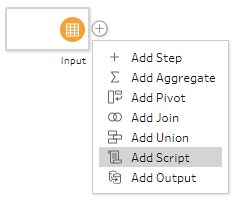## Getting Started with R Scripts in Tableau Prep

In the most recent release of Tableau Prep (2019.3), we have been treated to an extra connection option: Add Script.

This allows you to use an R or Python Script within your flow, which opens up a world of possibilities! Sometimes you need to learn to walk before you can run though, so the aim of this blog is to take you through the first steps to unlocking the power of R within Tableau Prep.

The first thing to understand is that you don’t actually write the script within Tableau Prep. Instead you connect to an R script through Rserve Server, which you need to set up within R.

R has a lot of functionality built in as standard, but it’s possible to access even more through packages:

The package that will allow us to set up an Rserve Server is called Rserve. By selecting this in the pop out window, R will automatically download it and we will see this text in the R console:

You will be prompted to select a CRAN mirror, which you will just select as the closest location to you.

Once Rserve has been selected in the pop out window, the following text will appear in the console:

All that remains is to call the downloaded function in the R console:

### Step 4: Connecting to Rserve in Tableau Prep

Now we have our Rserve Server, we will connect to it in the Script step in Tableau Prep:

As the name suggests, localhost is hosted locally on your laptop and port 6311 is the default port for plaintext Rserve Servers. We’re now ready to build an R Script!

### Step 5: Example function: Multiply a field by 2

When I said we were going to keep things basic, I meant it! For this first example we are simply going to take a field of data in Tableau Prep that contains the numbers 1 – 10 and multiply each of them by 2. To do this we need to build a script in R:

This will open a new window, where we can type the following code:

``````multiply<-function(n) {
return(2*n)
}``````

For those unfamiliar with R syntax, multiply is what we have defined as the function name in this example. The ‘<-‘ works like an ‘=’ sign to assign the purpose of multiply. The (n) is defining the variables that will be read into the function. There can be more than 1 variable (for instance if we had more than 1 field in our data) and these variables would be separated by commas.

In R, curly brackets act as the beginning and end of where a function will apply, similar to the way that you need an ‘end’ at the end of an if statement in a calculated field. The return then tells R what to output from the function, in this case multiplying every row of data by 2.

Note: If your function is changing the data structure (e.g. by adding a column) you will need some additional code at the end of your script to explain what data type it should be in Tableau Prep. I shan’t go into detail in this blog, but an example can be found here.

To test this code is working in R, we will want to save our script (creating a .R file), run it in the console and call the function on some example data:

You may notice that we haven’t defined what the variable n actually is. In the above test, I used the function on an integer, whereas when we use it in Tableau Prep, we would be using it on a data frame (i.e. a table). Either variable works with this function, as it is a simple multiplication, but this is not always the case.

Whilst tools like Tableau Prep will apply a calculated field on a row by row basis, R does not work in this way. It is a statistical software and you may not always be dealing with 2 dimensional tables, but rather multidimensional arrays. Therefore, it is sometimes necessary to use a for loop in order to tell R to deal with each row 1 at a time, as will be demonstrated in a future blog.

You will need to click browse to find the .R file that you saved earlier (which doesn’t have to have the same name as the function). Then you need to tell Tableau Prep what function it’s looking for, which in this case we named multiply.

Clearly we can see in the output that each of the numbers from 1 – 10 has been multiplied by 2 and so we have had success!

London

### One thought on “Getting Started with R Scripts in Tableau Prep”

1.Rolando Mirabal Hinojosa says:

Thanks! it worked like a blast, would be looking for the future blogs of r & prep